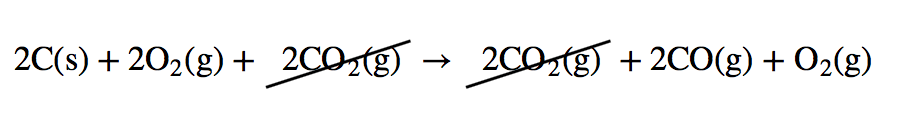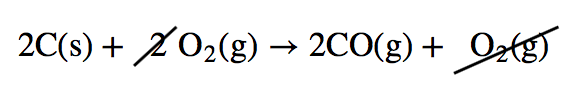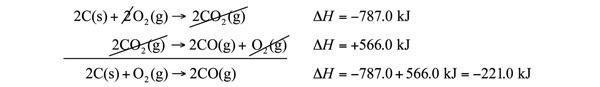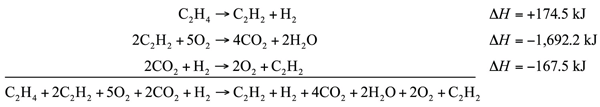## Hess’s Law

### Learning Objective

1. Learn how to combine chemical equations and their enthalpy changes.

Now that we understand that chemical reactions occur with a simultaneous change in energy, we can apply the concept more broadly. To start, remember that some chemical reactions are rather difficult to perform. For example, consider the combustion of carbon to make carbon monoxide:

2 C(s) + O2(g) → 2 CO(g) ΔH = ?

In reality, this is extremely difficult to do; given the opportunity, carbon will react to make another compound, carbon dioxide:

2 C(s) + O2(g) → 2 CO2(g) ΔH = −393.5 kJ

Is there a way around this? Yes. It comes from the understanding that chemical equations can be treated like algebraic equations, with the arrow acting like the equals sign. Like algebraic equations, chemical equations can be combined, and if the same substance appears on both sides of the arrow, it can be canceled out (much like a spectator ion in ionic equations). For example, consider these two reactions:

2 C(s) + 2 O2(g) → 2 CO2(g)
2 CO2(g) → 2 CO(g) + O2(g)

If we added these two equations by combining all the reactants together and all the products together, we would get

2 C(s) + 2 O2(g) + 2 CO2(g) → 2 CO2(g) + 2 CO(g) + O2(g)

We note that 2 CO2(g) appears on both sides of the arrow, so they cancel:We also note that there are 2 mol of O2 on the reactant side, and 1 mol of O2 on the product side. We can cancel 1 mol of O2 from both sides:What do we have left?

2 C(s) + O2(g) → 2 CO(g)

This is the reaction we are looking for! So by algebraically combining chemical equations, we can generate new chemical equations that may not be feasible to perform.

What about the enthalpy changes? Hess’s law states that when chemical equations are combined algebraically, their enthalpies can be combined in exactly the same way. Two corollaries immediately present themselves:

1. If a chemical reaction is reversed, the sign on ΔH is changed.
2. If a multiple of a chemical reaction is taken, the same multiple of the ΔH is taken as well.

What are the equations being combined? The first chemical equation is the combustion of C, which produces CO2:

2 C(s) + 2 O2(g) → 2 CO2(g)

This reaction is two times the reaction to make CO2 from C(s) and O2(g), whose enthalpy change is known:

C(s) + O2(g) → CO2(g) ΔH = −393.5 kJ

According to the first corollary, the first reaction has an energy change of two times −393.5 kJ, or −787.0 kJ:

2 C(s) + 2 O2(g) → 2 CO2(g) ΔH = −787.0 kJ

The second reaction in the combination is related to the combustion of CO(g):

2 CO(g) + O2(g) → 2 CO2(g) ΔH = −566.0 kJ

The second reaction in our combination is the reverse of the combustion of CO. When we reverse the reaction, we change the sign on the ΔH:

2 CO2(g) → 2 CO(g) + O2(g) ΔH = +566.0 kJ

Now that we have identified the enthalpy changes of the two component chemical equations, we can combine the ΔH values and add them:Hess’s law is very powerful. It allows us to combine equations to generate new chemical reactions whose enthalpy changes can be calculated, rather than directly measured.

### Example 10

Determine the enthalpy change of

C2H4 + 3 O2 → 2 CO2 + 2 H2O ΔH = ?

from these reactions:

C2H2 + H2 → C2H4 ΔH = −174.5 kJ
2 C2H2 + 5 O2 → 4 CO2 + 2 H2O ΔH = −1,692.2 kJ
2 CO2 + H2 → 2 O2 + C2H2 ΔH = −167.5 kJ

Solution

We will start by writing chemical reactions that put the correct number of moles of the correct substance on the proper side. For example, our desired reaction has C2H4 as a reactant, and only one reaction from our data has C2H4. However, it has C2H4 as a product. To make it a reactant, we need to reverse the reaction, changing the sign on the ΔH:

C2H4 → C2H2 + H2 ΔH = +174.5 kJ

We need CO2 and H2O as products. The second reaction has them on the proper side, so let us include one of these reactions (with the hope that the coefficients will work out when all our reactions are added):

2 C2H2 + 5 O2 → 4 CO2 + 2 H2O ΔH = −1,692.2 kJ

We note that we now have 4 mol of CO2 as products; we need to get rid of 2 mol of CO2. The last reaction has 2CO2 as a reactant. Let us use it as written:

2 CO2 + H2 → 2 O2 + C2H2 ΔH = −167.5 kJ

We combine these three reactions, modified as stated:What cancels? 2 C2H2, H2, 2 O2, and 2 CO2. What is left is

C2H4 + 3 O2 → 2 CO2 + 2H2O

which is the reaction we are looking for. The ΔH of this reaction is the sum of the three ΔH values:

ΔH = +174.5 − 1,692.2 − 167.5 = −1,685.2 kJ

Test Yourself

Given the thermochemical equations

Pb + Cl2 → PbCl2 ΔH = −223 kJ
PbCl2 + Cl2 → PbCl4 ΔH = −87 kJ

determine ΔH for

2 bCl2 → Pb + PbCl4

+136 kJ

### Key Takeaway

• Hess’s law allows us to combine reactions algebraically and then combine their enthalpy changes the same way.

### Exercises

1. Define Hess’s law.

2. What does Hess’s law require us to do to the ΔH of a thermochemical equation if we reverse the equation?

3. If the ΔH for

C2H4 + H2 → C2H6

is −65.6 kJ, what is the ΔH for this reaction?

C2H6 → C2H4 + H2

4. If the ΔH for

2 Na + Cl2 → 2NaCl

is −772 kJ, what is the ΔH for this reaction:

2 NaCl → 2 Na + Cl2

5. If the ΔH for

C2H4 + H2 → C2H6

is −65.6 kJ, what is the ΔH for this reaction?

2 C2H4 + 2 H2 → 2 C2H6

6. If the ΔH for

2 C2H6 + 7 O2 → 4 CO2 + 6 H2O

is −2,650 kJ, what is the ΔH for this reaction?

6 C2H6 + 21 O2 → 12 CO2 + 18 H2O

7. The ΔH for

C2H4 + H2O → C2H5OH

is −44 kJ. What is the ΔH for this reaction?

2 C2H5OH → 2 C2H4 + 2 H2O

8. The ΔH for

N2 + O2 → 2 NO

is 181 kJ. What is the ΔH for this reaction?

NO → 1/2 N2 + 1/2 O2

9. Determine the ΔH for the reaction

Cu + Cl2 → CuCl2

given these data:

2 Cu + Cl2 → 2 CuCl ΔH = −274 kJ
2 CuCl + Cl2 → 2 CuCl2 ΔH = −166 kJ

10. Determine ΔH for the reaction

2 CH4 → 2 H2 + C2H4

given these data:

CH4 + 2 O2 → CO2 + 2 H2O ΔH = −891 kJ
C2H4 + 3 O2 → 2 CO2 + 2 H2O ΔH = −1,411 kJ
2 H2 + O2 → 2 H2O ΔH = −571 kJ

11. Determine ΔH for the reaction

Fe2(SO4)3 → Fe2O3 + 3SO3

given these data:

4 Fe + 3O2 → 2 Fe2O3 ΔH = −1,650 kJ
2 S + 3 O2 → 2 SO3 ΔH = −792 kJ
2 Fe + 3 S + 6 O2 → Fe2(SO4)3 ΔH = −2,583 kJ

12. Determine ΔH for the reaction

CaCO3 → CaO + CO2

given these data:

2 Ca + 2 C + 3 O2 → 2 CaCO3 ΔH = −2,414 kJ
C + O2 → CO2 ΔH = −393.5 kJ
2 Ca + O2 → 2 CaO ΔH = −1,270 kJ

1.

If chemical equations are combined, their energy changes are also combined.

3.

ΔH = 65.6 kJ

5.

ΔH = −131.2 kJ

7.

ΔH = 88 kJ

9.

ΔH = −220 kJ

11.

ΔH = 570 kJ